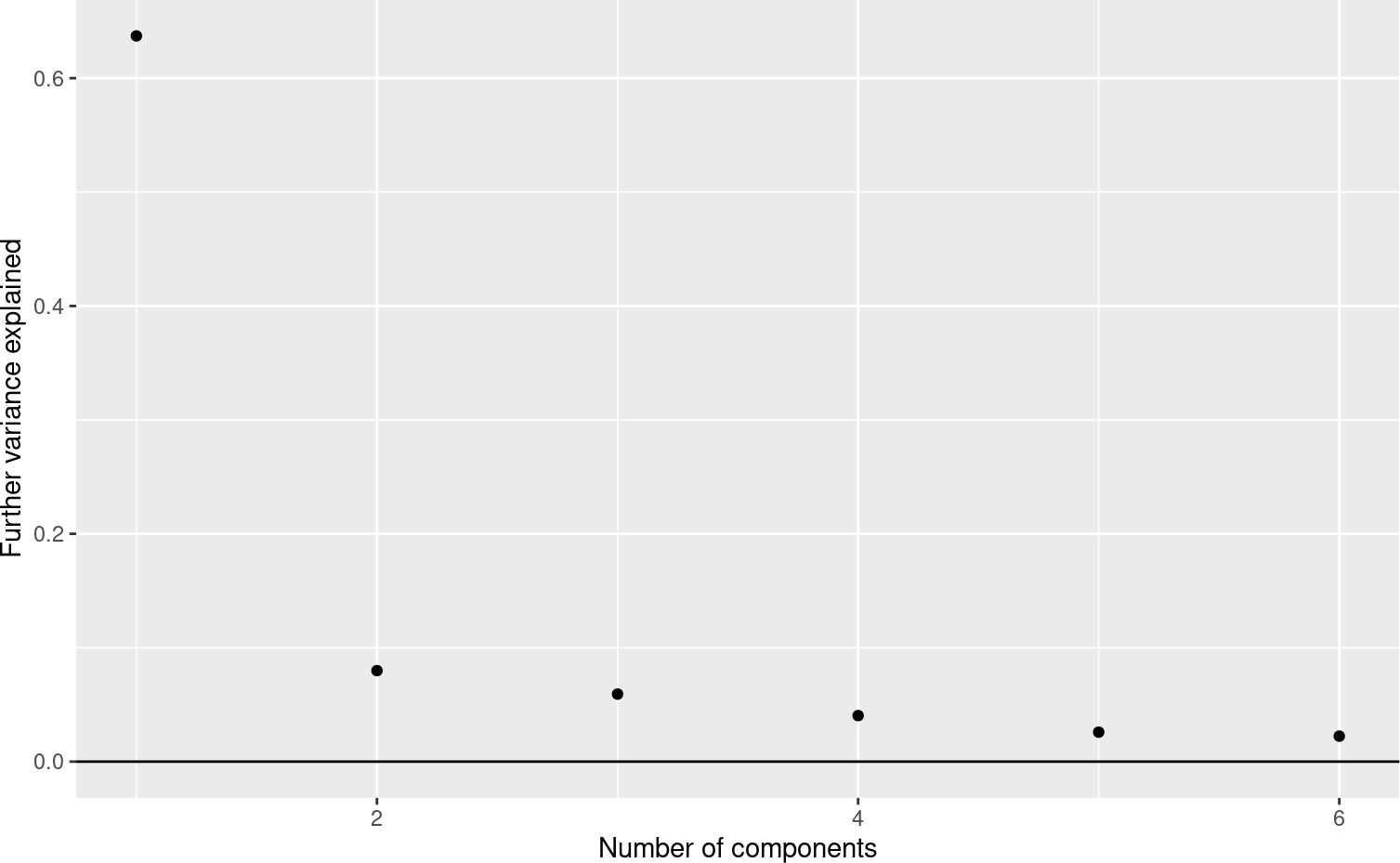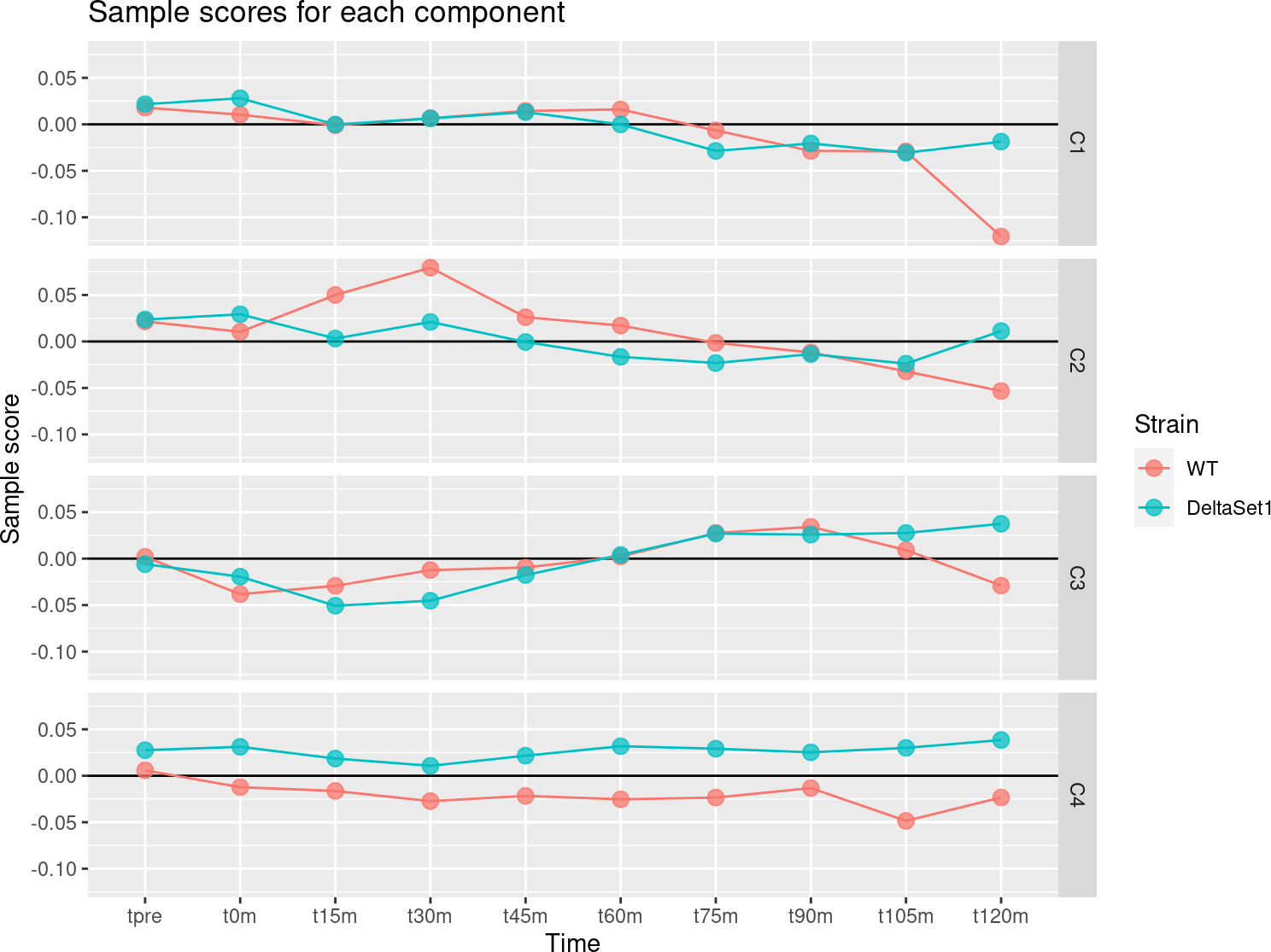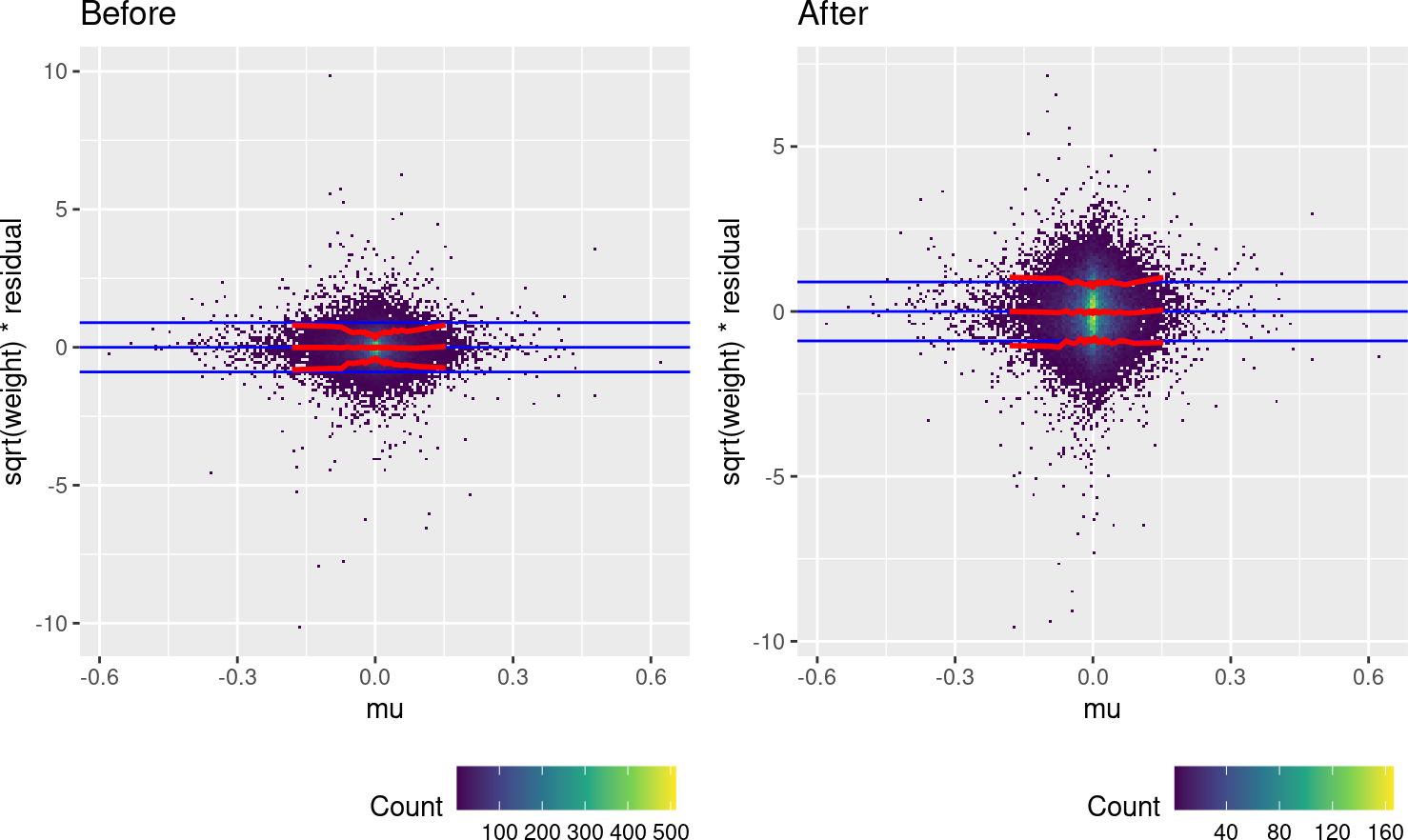# Contents

APA sites can be detected using the PAT-Seq protocol. This protocol produces 3’-end focussed reads. We examine GSE83162. This is a time-series experiment in which two strains of yeast are released into synchronized cell cycling and observed through two cycles. Yeast are treated with $$\alpha$$-factor, which causes them to stop dividing in antici… pation of a chance to mate. When the $$\alpha$$-factor is washed away, they resume cycling.

# 1 Shift score definition

Each gene has several APA sites, ordered from furthest upstrand to furthest downstrand. For each sample, we have a read count at each site.

For each gene:

We define the “shift” of a particular sample relative to all reads from all samples.

A “shift” score is first assigned to each site, being the proportion of all reads upstrand of the site minus the proportion of all reads downstrand of it (i.e. an average over all reads where upstrand reads are 1, downstrand reads are -1 and reads from the site itself are 0). The measurement for each sample is then an average over the site score for each read. The weight is the number of reads.

Shifts scores range from -1 to 1, and summarize whether upstrand (more negative) or downstrand (more positive) sites are being favoured. The weighted average score is zero.

The weights are the number of reads, however for a randomly chosen read we can estimate its variance based on the number of reads at each site and the site scores. (This estimate of variance includes any biological signal, so it’s not exactly a residual variance.) This is stored in the rowData() of the weitrix, and can be used to further calibrate weights. We prefer to defer this sort of calibration until after we’ve discoverd components of variation, as it tends to give high weight to genes with little APA. There are clearly some alternative choices to how weighting could be performed, and we hope the weitrix package gives you basic building blocks with which you can experiment!

library(tidyverse)    # ggplot2, dplyr, etc
library(patchwork)    # side-by-side ggplots
library(reshape2)     # melt()
library(weitrix)      # Matrices with precision weights

# Produce consistent results
set.seed(12345)

# BiocParallel supports multiple backends.
# If the default hangs or errors, try others.
# The most reliable backed is to use serial processing
BiocParallel::register( BiocParallel::SerialParam() )
peaks <- system.file("GSE83162", "peaks.csv.gz", package="weitrix") %>%

counts <- system.file("GSE83162", "peak_count.csv.gz", package="weitrix") %>%
column_to_rownames("name") %>%
as.matrix()

genes <- system.file("GSE83162", "genes.csv.gz", package="weitrix") %>%
column_to_rownames("name")

samples <- data.frame(sample=I(colnames(counts))) %>%
extract(sample, c("strain","time"), c("(.+)-(.+)"), remove=FALSE) %>%
mutate(
strain=factor(strain,unique(strain)),
time=factor(time,unique(time)))
rownames(samples) <- samples$sample groups <- dplyr::select(peaks, group=gene_name, name=name) # Note the order of this data frame is important samples ## sample strain time ## WT-tpre WT-tpre WT tpre ## WT-t0m WT-t0m WT t0m ## WT-t15m WT-t15m WT t15m ## WT-t30m WT-t30m WT t30m ## WT-t45m WT-t45m WT t45m ## WT-t60m WT-t60m WT t60m ## WT-t75m WT-t75m WT t75m ## WT-t90m WT-t90m WT t90m ## WT-t105m WT-t105m WT t105m ## WT-t120m WT-t120m WT t120m ## DeltaSet1-tpre DeltaSet1-tpre DeltaSet1 tpre ## DeltaSet1-t0m DeltaSet1-t0m DeltaSet1 t0m ## DeltaSet1-t15m DeltaSet1-t15m DeltaSet1 t15m ## DeltaSet1-t30m DeltaSet1-t30m DeltaSet1 t30m ## DeltaSet1-t45m DeltaSet1-t45m DeltaSet1 t45m ## DeltaSet1-t60m DeltaSet1-t60m DeltaSet1 t60m ## DeltaSet1-t75m DeltaSet1-t75m DeltaSet1 t75m ## DeltaSet1-t90m DeltaSet1-t90m DeltaSet1 t90m ## DeltaSet1-t105m DeltaSet1-t105m DeltaSet1 t105m ## DeltaSet1-t120m DeltaSet1-t120m DeltaSet1 t120m head(groups, 10) ## # A tibble: 10 x 2 ## group name ## <chr> <chr> ## 1 YDR533C peak1653 ## 2 YDR533C peak1652 ## 3 YDR529C peak1648 ## 4 YDR529C peak1647 ## 5 snR84 peak1646 ## 6 snR84 peak1645 ## 7 snR84 peak1644 ## 8 YDR524C-B peak1643 ## 9 YDR524C-B peak1642 ## 10 YDR500C peak1629 counts[1:10,1:5] ## WT-tpre WT-t0m WT-t15m WT-t30m WT-t45m ## peak3910 58 24 46 54 41 ## peak3911 25 9 24 28 18 ## peak3912 7 9 10 13 18 ## peak4139 30 4 7 12 13 ## peak4140 8 1 1 8 2 ## peak4141 55 7 13 19 20 ## peak8533 37 35 42 64 47 ## peak8534 38 50 44 53 36 ## peak5235 16 4 2 7 4 ## peak5236 14 5 2 5 4 A “shift” weitrix is constructed based on a matrix of site-level counts, plus a data frame grouping sites into genes. The order of this data frame is important, earlier sites are considered upstrand of later sites. wei <- counts_shift(counts, groups) ## Calculating shifts in 1 blocks colData(wei) <- cbind(colData(wei), samples) rowData(wei)$symbol <- genes$symbol[match(rownames(wei), rownames(genes))] Having obtained a weitrix, everthing discussed for the poly(A) tail length example is applicable here as well. We will only perform a brief exploratory analysis here. # 3 Exploratory analysis ## 3.1 Components of variation We can look for components of variation. comp_seq <- weitrix_components_seq(wei, p=6, design=~0) components_seq_screeplot(comp_seq)Pushing a point somewhat, we examine four components. comp <- comp_seq[] matrix_long(comp$col, row_info=samples, varnames=c("sample","component")) %>%
ggplot(aes(x=time, y=value, color=strain, group=strain)) +
geom_hline(yintercept=0) +
geom_line() +
geom_point(alpha=0.75, size=3) +
facet_grid(component ~ .) +
labs(title="Sample scores for each component", y="Sample score", x="Time", color="Strain")## 3.2 Calibration

Weights can be calibrated. Ideally the weights should be 1 over the residual variance. We will fit a gamma GLM with log link function to the squared residuals, and use the predictions from this to produce better weights. This model uses the per_read_var information present in the rowData, as well as applying a non-linear transformation to the existing weights.

cal <- weitrix_calibrate_all(
wei,
design = comp,
trend_formula = ~log(per_read_var)+well_knotted_spline(log2(weight),3))
## log2(weight) range  0.00000 19.70383 knots  4.863982  7.206426 10.386132
metadata(cal)$weitrix$all_coef
##                           (Intercept)                     log(per_read_var)
##                              0.209536                              1.185355
## well_knotted_spline(log2(weight), 3)1 well_knotted_spline(log2(weight), 3)2
##                             -4.692578                             -5.234432
## well_knotted_spline(log2(weight), 3)3 well_knotted_spline(log2(weight), 3)4
##                             -9.261400                             -6.398811

A mean-variance trend is visible in the uncalibrated weighted residuals. Calibration has removed this, by making use of the information in per_read_var.

(weitrix_calplot(wei, comp, covar=mu) + labs(title="Before")) +
(weitrix_calplot(cal, comp, covar=mu) + labs(title="After"))We can look at the calibration to per_read_var directly.

(weitrix_calplot(wei, comp, covar=per_read_var) + labs(title="Before")) +
(weitrix_calplot(cal, comp, covar=per_read_var) + labs(title="After"))